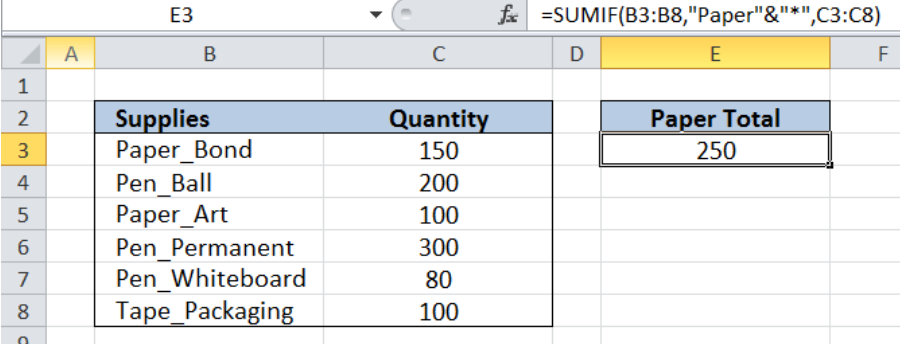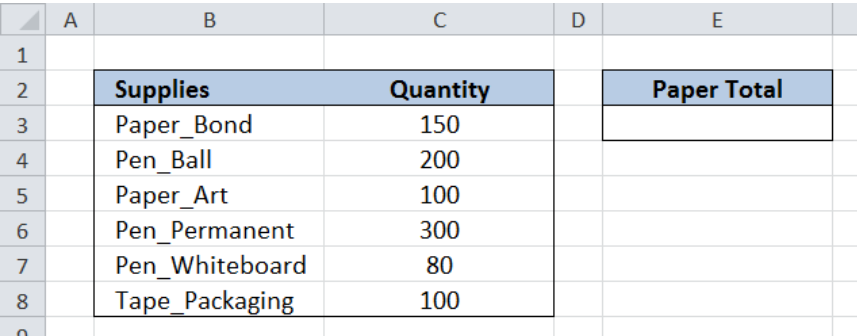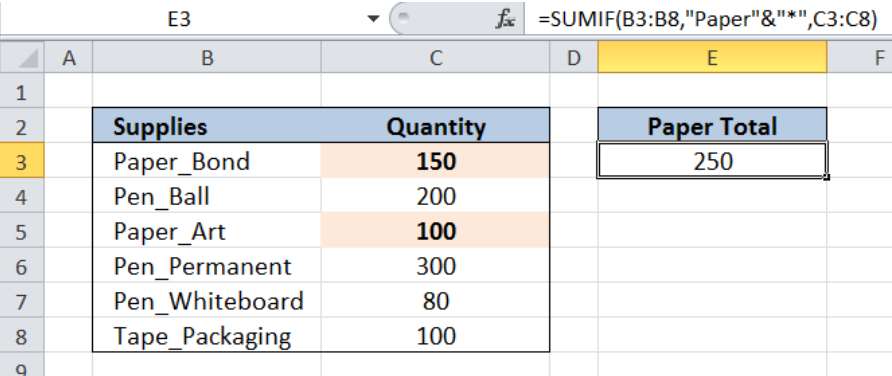Get instant live expert help with Excel or Google Sheets“My Excelchat expert helped me in less than 20 minutes, saving me what would have been 5 hours of work!”

#### Post your problem and you’ll get Expert help in seconds.

Your message must be at least 40 characters
Our professional Expert are available now. Your privacy is guaranteed.

# Sum If Begins With

While working with Excel, we are able to sum values in a data set based on a given criteria by using the SUMIF function.  SUMIF sums the values in a specified range based on a given condition.  This step by step tutorial will assist all levels of Excel users in summing values that begin with a specific text.Figure 1. Final result of the SUMIF function

Final Formula: `=SUMIF(B3:B8,"Paper"&"*",C3:C8)`

## Syntax of the SUMIF Function

SUMIF sums the values in a specified range, based on one given criteria

### Syntax

`=SUMIF(range,criteria, [sum_range])`

Where

• Range: the data range that will be evaluated using the criteria
• Criteria: the criteria or condition that determines which cells will be added
• Sum_range: the cells that will be added; if left blank, “sum_range” = “range” which means that the range of data that will be added is the same range of data evaluated

## Setting up the Data

Our table has two columns: Supplies (column B) and Quantity (column C).  We want to get the total quantity for Paper supplies and record it in cell E3.Figure 2. Sample data for the SUMIF example

## Sum Quantity if Supplies Begin with Paper

We want to sum all values in column C (Quantity) if the cells in column B (Supplies) begin with “Paper”.  Here are the steps:

Step 1. Select cell E3

Step 2. Enter the formula: `=SUMIF(B3:B8,"Paper"&"*",C3:C8)`

Step 3. Press Enter

The range for the data we want to evaluate is B3:B8.  The criteria is “Paper”&”*” which translates to “ Paper* ”.  The asterisk *” means that there could be any number of characters after the word “Paper”.  The sum_range is C3:C8 which is the column for “Quantity” that we want to add.  As a result, the SUMIF function returns 250, which is the sum of the quantity of “Paper_Bond” and “Paper_Art”.Figure 3. Using the SUMIF function to sum values of supplies that begin with “Paper”

Most of the time, the problem you will need to solve will be more complex than a simple application of a formula or function. If you want to save hours of research and frustration, try our live Excelchat service! Our Excel Experts are available 24/7 to answer any Excel question you may have. We guarantee a connection within 30 seconds and a customized solution within 20 minutes.

### Did this post not answer your question? Get a solution from connecting with the expert.Another blog reader asked this question today on Excelchat:
Solution examplesamending a formula so that there is another criteria and if it does not meet this criteria then we stick with the original criteria
Solved by A. B. in 40 minsI need Column D to = Column C - Column A only if Column B has a 'Date' entered.
Solved by I. B. in 11 minsHello, I need help trying to create a sum of numerical values on a spreadsheet in which the only values that apply to the sum are when the status of another cell is a certain value (e.g. value is not in sum when status: closed, value is in sum when status: open)
Solved by T. W. in 18 minsI am looking for a formula to SUM column L, if Column A matched the relevant date and column C matches the name
Solved by Z. Y. in 16 minsI need a formula that is essentially saying IF this range of cells contain a certain name, add the number in another cell on the same row, and them sum that number in a different cell..!
Solved by O. H. in 44 mins## Subscribe to Excelchat.coAnother blog reader asked this question today on Excelchat: